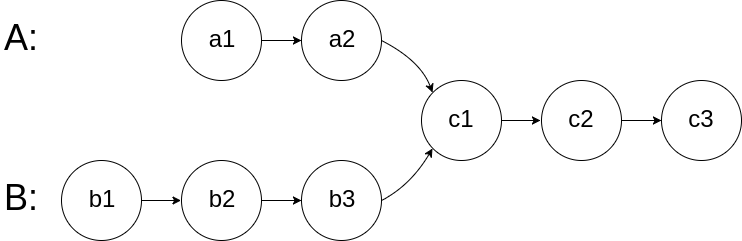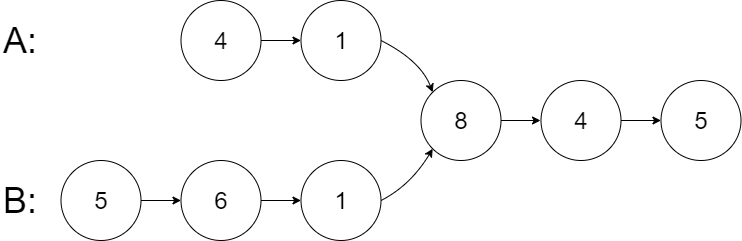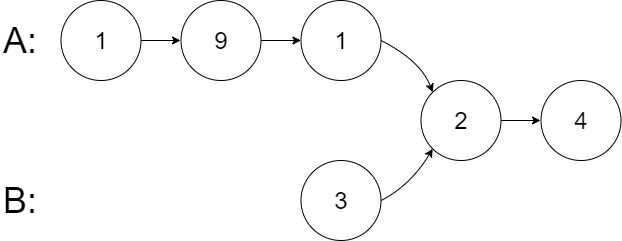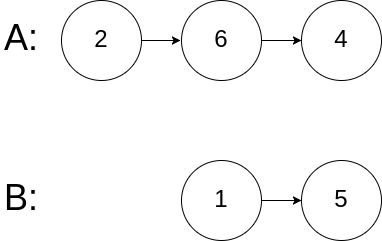### 描述

Given the heads of two singly linked-lists headA and headB, return the node at which the two lists intersect. If the two linked lists have no intersection at all, return null.

For example, the following two linked lists begin to intersect at node c1:The test cases are generated such that there are no cycles anywhere in the entire linked structure.

Note that the linked lists must retain their original structure after the function returns.

Custom Judge:

The inputs to the judge are given as follows (your program is not given these inputs):

• intersectVal - The value of the node where the intersection occurs. This is 0 if there is no intersected node.
• listA - The first linked list.
• listB - The second linked list.
• skipA - The number of nodes to skip ahead in listA (starting from the head) to get to the intersected node.
• skipB - The number of nodes to skip ahead in listB (starting from the head) to get to the intersected node.

The judge will then create the linked structure based on these inputs and pass the two heads, headA and headB to your program. If you correctly return the intersected node, then your solution will be accepted.

Example 1:Example 2:Example 3:Constraints:

• The number of nodes of listA is in the m.
• The number of nodes of listB is in the n.
• 0 <= m, n <= 3 * 104
• 1 <= Node.val <= 105
• 0 <= skipA <= m
• 0 <= skipB <= n
• intersectVal is 0 if listA and listB do not intersect.
• intersectVal == listA[skipA] == listB[skipB] if listA and listB intersect.

Follow up: Could you write a solution that runs in O(n) time and use only O(1) memory?